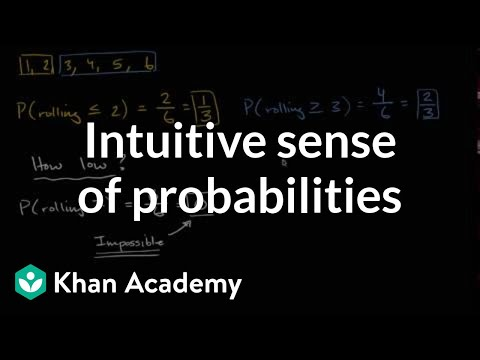Video

# Intro to theoretical probability (Full video)

Description: We give you an introduction to probability through the example of flipping a quarter and rolling a die. Created by Sal Khan. And so when I talk about a fair coin, I mean that it has an equal chance of landing on one side or another. I'm going to flip a coin. And I want to know what is the probability of getting heads.

### Other videos you might be interested in### Intuitive sense of probabilities (Full video)